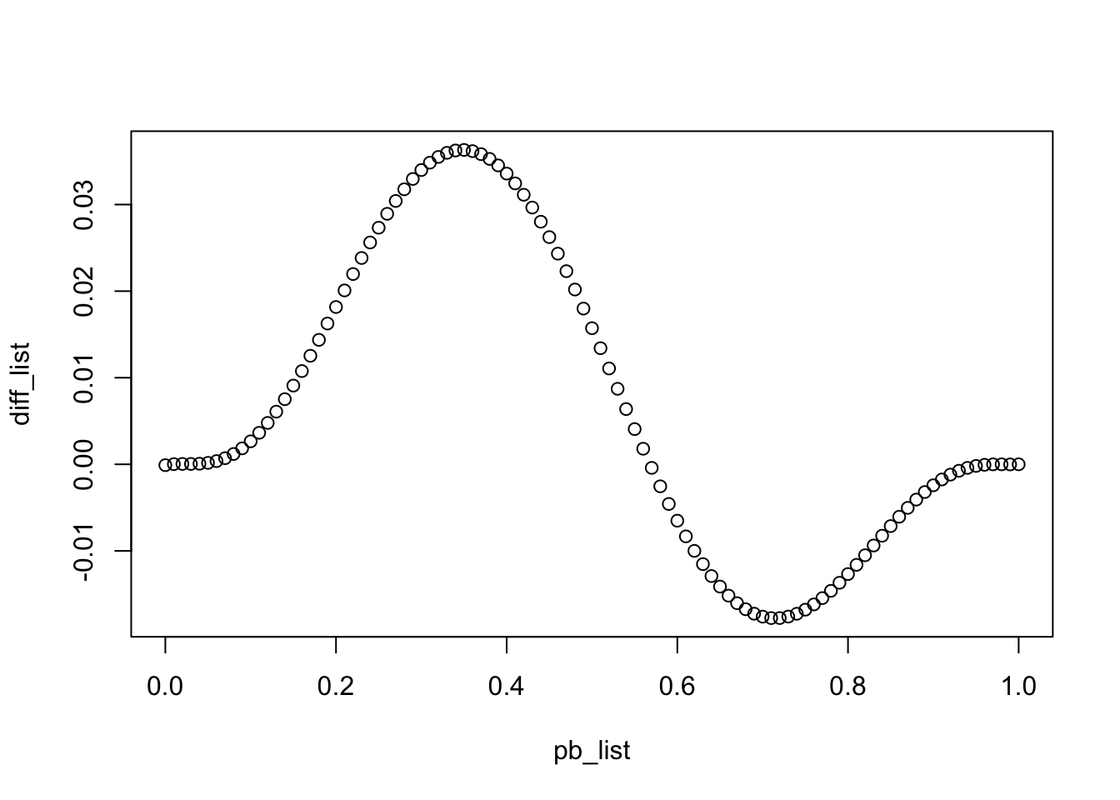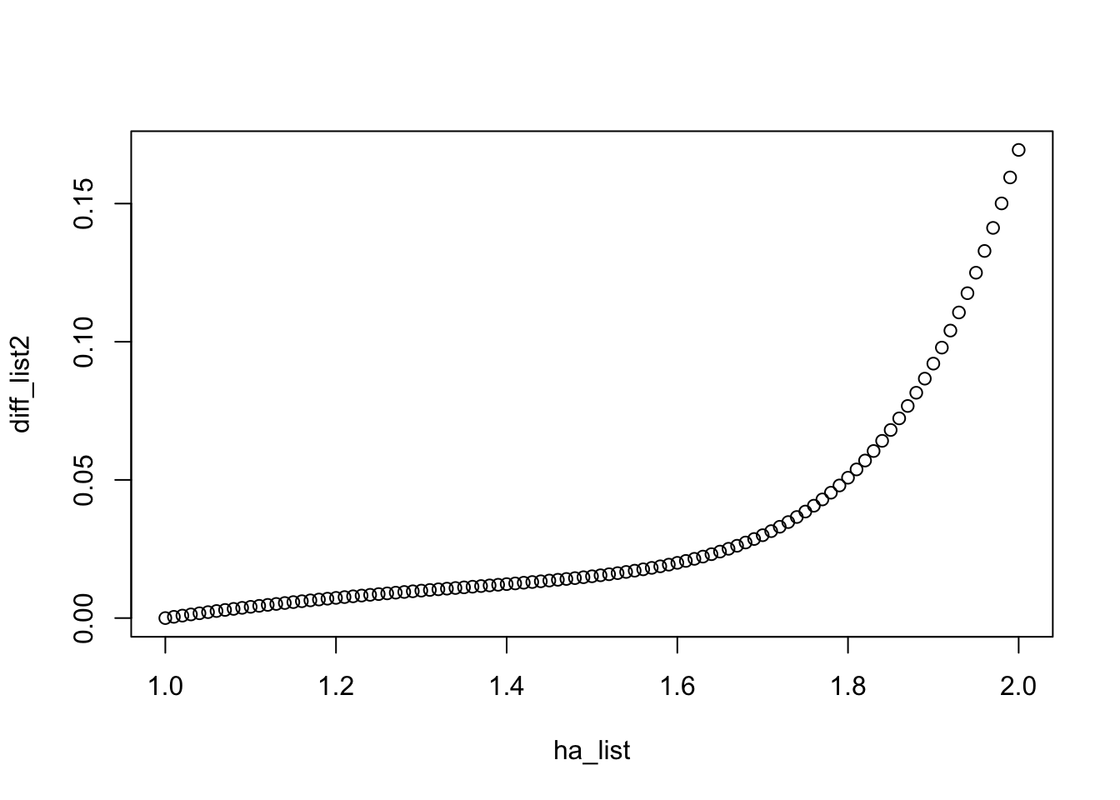# If home-field advantage exists, how much of an impact does it have on winning the world series?

## Probability and Statistical Inference - 04

Posted by Zekun on September 12, 2019

# IntroductionIn team sports, the term home advantage – also called home ground, home field, home-field advantage, home court, home-court advantage, defender’s advantage or home-ice advantage – describes the benefit that the home team is said to gain over the visiting team. This benefit has been attributed to psychological effects supporting fans have on the competitors or referees; to psychological or physiological advantages of playing near home in familiar situations; to the disadvantages away teams suffer from changing time zones or climates, or from the rigors of travel; … In baseball, in particular, the difference may also be the result of the home team having been assembled to take advantage of the idiosyncrasies of the home ballpark, such as the distances to the outfield walls; most other sports are played in standardized venues. From Wikipedia - Home advantage

This time we will also talk about the competitions between the Braves and the Yankee, and the table below has the two possible schedules for each game of the series. (NYC = New York City, ATL = Atlanta)

Overall advantage Game 1 Game 2 Game 3 Game 4 Game 5 Game 6 Game 7
Braves ATL ATL NYC NYC NYC ATL ATL
Yankees NYC NYC ATL ATL ATL NYC NYC

Let PB be the probability that the Braves win a single head-to-head match-up with the Yankees, under the assumption that home-field advantage doesn’t exist. Let PBH denote the probability that the Braves win a single head-to-head match-up with the Yankees as the home team (H for home). Let PBA denote the probability that the Braves win a single head-to-head match-up with the away team (A for away).

ATL PB PBH = PB $\times$ 1.1
NYC PB PBA = 1 - (1 - PB)$\times$ 1.1
library(dplyr)
library(data.table)


Now let’s look at the questions.

# Questions

## 1. Compute analytically the probability that the Braves win the world series when the sequence of game locations is {NYC, NYC, ATL, ATL, ATL, NYC, NYC}. Calculate the probability with and without home field advantage when PB = 0.55. What is the difference in probabilities?

First, load the .csv file to make sure what’s the situations we need to calculate, which represents the data we will generate later.

# Get all possible outcomes


And we need to define a sequence of game locations. This time, the sequence should be {NYC, NYC, ATL, ATL, ATL, NYC, NYC}. As a result of that the Braves is an Atlanta team, we use 1 to represent Atlanta and use 0 to represent NYC.

# Home field indicator
hfi <- c(0,0,1,1,1,0,0) #{NYC, NYC, ATL, ATL, ATL, NYC, NYC}


Now we use 0.55 to define the PB and generate other probabilities by PB as we talked before.

# P_B
pb <- 0.55
pba <- 1 - (1 - pb) * advantage_multiplier


In this part, we will use the parameters we defined before. In every row of data.table, we use the different probabilities which influenced by home-field advantage to calculate the overall probabilities of each situation.

# Calculate the probability of each possible outcome
apo[, p := NA_real_] # Initialize new column in apo to store prob
for(i in 1:nrow(apo)){
prob_game <- rep(1, 7)
for(j in 1:7){
p_win <- ifelse(hfi[j], pbh, pba)
prob_game[j] <- case_when(
apo[i,j,with=FALSE] == "W" ~ p_win
, apo[i,j,with=FALSE] == "L" ~ 1 - p_win
, TRUE ~ 1
)
}
apo[i, p := prod(prob_game)] # Data.table syntax
}


Then we output the probability that the Braves wins the World Series under the influence of home field advantage.

# Probability of overall World Series outcomes
p_home <- purrr::flatten_dbl(apo[, sum(p), overall_outcome][1,2])
p_home


The probability is r p_home.

Then we can calculate the probability when there is no home field advantage.

p_nohome <- 1 - pbinom(3, 7, 0.55)
p_nohome


The probability is r p_nohome. Definitely, when home-field advantage exists, the probability that the Braves win the World Series is lower, r p_home, than the probability without home-field advantage. Given PB=0.55, the probability of the Braves winning the World Series with a home field advantage r (p_home-p_nohome)*100 a less likely than the probability of the Braves winning the World Series without a home-field advantage.

## 2. Calculate the same probabilities as the previous question by simulation.

In this part, we will use simulation to test the probability.

Given the location sequence, we use different winning probabilities of the head to head games and randomly generate the result of each game. Repeat the process 100000 times, we can get the approximate solution of the probability with home field advantage influence.

set.seed(314)
sml_list_h <- rep(NA, 100000)
for (i in seq_along(sml_list_h)){
round <- rep(NA,7)
for(j in 1:7){
p_win <- ifelse(hfi[j], pbh, pba)
round[j] <- rbinom(1,1,p_win)
}
sml_list_h[i] <- ifelse(sum(round)>=4, 1, 0)
}
mean_sml_h <- mean(sml_list_h)
mean_sml_h


Now we can get the approximate solution, r mean_sml_h, which is a little different from r p_home.

Now, let’s simulate the situation without home-field advantage influence. It’s easy because we only need to make p_win as a constant value.

set.seed(314)
sml_list_nh <- rep(NA, 100000)
for (i in seq_along(sml_list_nh)){
round <- rep(NA,7)
for(j in 1:7){
p_win <- 0.55
round[j] <- rbinom(1,1,p_win)
}
sml_list_nh[i] <- ifelse(sum(round)>=4, 1, 0)
}
mean_sml_nh <- mean(sml_list_nh)
mean_sml_nh


## 3. What is the absolute and relative error for your simulation in the previous question?

Absolute error = $$|p̂−p|$$

Relative error = $$|p̂−p|/p$$.

abs_error_h <- abs(mean(sml_list_h) - p_home)
rel_error_h <- abs(mean(sml_list_h) - p_home)/mean(sml_list_h)
abs_error_h
rel_error_h


Therefore, given home-field advantage the absolute error is r abs_error_h. The relative error is r rel_error_h.

abs_error_nh <- abs(mean(sml_list_nh) - p_nohome)
rel_error_nh <- abs(mean(sml_list_nh) - p_nohome)/mean(sml_list_nh)
abs_error_nh
rel_error_nh


Therefore, given no home-field advantage the absolute error is r abs_error_nh. The relative error is r rel_error_nh.

## Bonus 1. Does the difference in probabilites (with vs without home field advantage) depend on PB?

The process is similar to the answer of question 1.

We can create some lists to save the different PBs, the probabilities of win the World Series with or without home-field advantage, and the difference between these two situations given different PB.

Then given every PB, calculate these values every time.

pb_list <- seq(0,1,0.01)
ph_win_list <- seq_along(pb_list)
pnh_win_list <- ph_win_list
diff_list <- ph_win_list
for (p in seq_along(pb_list)) {
pb <- pb_list[p]
pba <- 1 - (1 - pb) * advantage_multiplier

pnh_win_list[p] <- 1 - pbinom(3, 7, pb_list[p])

# Calculate the probability of each possible outcome
apo[, p := NA_real_] # Initialize new column in apo to store prob
for(i in 1:nrow(apo)){
prob_game <- rep(1, 7)
for(j in 1:7){
p_win <- ifelse(hfi[j], pbh, pba)
prob_game[j] <- case_when(
apo[i,j,with=FALSE] == "W" ~ p_win
, apo[i,j,with=FALSE] == "L" ~ 1 - p_win
, TRUE ~ 1
)
}
apo[i, p := prod(prob_game)] # Data.table syntax
}
ph_win_list[p] <- purrr::flatten_dbl(apo[, sum(p), overall_outcome][,2])
diff_list[p] <- pnh_win_list[p] - ph_win_list[p]
}


Then, plot a graph to show the relationship between PB and differences.

plot(x= pb_list, y=diff_list)Obviously, the relationship between PB and differences is a compound function containing trigonometric functions.

And the format might be a trigonometric function times a function, that when going to the start and the end of one period approximate 0, otherwise approximate 1. By the way, the period should be 1.

There are some examples. $$y = A \cdot sin(B \cdot x + C) \\ y = A \cdot sin(B \cdot x + C) \cdot |D \cdot sin(\pi \cdot x + E)| \\ y = A \cdot sin(B \cdot x + C) \cdot ( D \cdot sin(\pi \cdot x + E))^2 \\ y = Ax^3 + Bx^2y + Cxy^2+Dy^3 +Ex^2+Fxy+Gy^2+Hx+Iy+J$$ Unfortunately, after these functions I listed cannot regress to the graph we got.

## Bonus 2. Does the difference in probabilites (with vs without home field advantage) depend on the advantage factor? (The advantage factor in PBH and PBA is the 1.1 multiplier that results in a 10% increase for the home team.)

In this question, the process is similar to Bonus1. We only need to change the sequence content from PB to the home-field advantage factor and make PB be a constant(0.55). Therefore, we will use ha_list to save the sequence.

ha_list <- seq(1,2,0.01)
ph_win_list <- seq_along(ha_list)
pnh_win_list <- ph_win_list
diff_list2 <- ph_win_list
for (p in seq_along(ha_list)) {
pb <- 0.55
pba <- 1 - (1 - pb) * advantage_multiplier

pnh_win_list[p] <- 1 - pbinom(3, 7, 0.55)

# Calculate the probability of each possible outcome
apo[, p := NA_real_] # Initialize new column in apo to store prob
for(i in 1:nrow(apo)){
prob_game <- rep(1, 7)
for(j in 1:7){
p_win <- ifelse(hfi[j], pbh, pba)
prob_game[j] <- case_when(
apo[i,j,with=FALSE] == "W" ~ p_win
, apo[i,j,with=FALSE] == "L" ~ 1 - p_win
, TRUE ~ 1
)
}
apo[i, p := prod(prob_game)] # Data.table syntax
}
ph_win_list[p] <- purrr::flatten_dbl(apo[, sum(p), overall_outcome][,2])
diff_list2[p] <- pnh_win_list[p] - ph_win_list[p]
}


Let’s look at the graph. Obviously, when the home-field advantage factor is increasing, the difference in probabilities between with and without home filed advantage will increase too.

plot(x= ha_list, y=diff_list2)